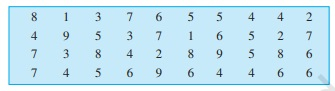# In a Mathematics test, the following marks were obtained by 40 students. Arrange these marks in a table using tally marks.(a) Find how many students obtained marks equal to or more than $7 .$(b) How many students obtained marks below 4?"

To do:

We have to find

(a) The number of students who obtained marks equal to or more than $7 .$

(b) The number of students who obtained marks below 4.
Solution:

 Marks Tally Marks Number of students 1 || 2 2 ||| 3 3 ||| 3 4 |||| || 7 5 |||| | 6 6 |||| || 7 7 |||| 5 8 |||| 4 9 ||| 3

(a) The number of students who obtained marks equal to or more than $7=$ The number of students who obtained 7 marks $+$ The number of students who obtained 8 marks $+$ The number of students who obtained 9 marks

$=5+4+3$

$=12$

(b) The number of students who obtained marks below $4=$ The number of students who obtained 3 marks $+$ The number of students who obtained 2 marks $+$ The number of students who obtained 1 mark

$=2+3+3$

$=8$